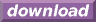#Calcute: freeware calculator

## Hyperbolic Arccosecant (hyperbolic arc-cosecant, hyperbolic inverse cosecant, acsch)

The hyperbolic arc-cosecant is the inverse of the hyperbolic cosecant function. Calcute implements it with the acsch function. The inverse hyperbolic cosecant of a value x is the value y for which the hyperbolic cosecant of y is x. In other words if y = acsch(x) then x = csch(y).

```acsch(1)
0.88137358702
```

The hyperbolic arc-cosecant function is undefined at 0.

In some mathematical texts and hand-held calculators, the notation csch-1 is sometimes used to represent the hyperbolic arc-cosecant function. This is an unfortunate notational choice since it could also stand for the multiplicative inverse 1/csch, which is a different function.

This function is sometimes called the "area hyperbolic cosecant". Another abbreviation sometimes used to represent it is "arccsch".